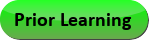Feb 06, 2023
2021-2022 College Catalog
 Select a Catalog 2022-2023 College Catalog 2021-2022 College Catalog [ARCHIVED CATALOG] 2020-2021 College Catalog [ARCHIVED CATALOG] 2019-2020 College Catalog [ARCHIVED CATALOG] DRAFT 2023-2024 College Catalog DRAFT [ARCHIVED CATALOG]
 HELP 2021-2022 College Catalog [ARCHIVED CATALOG] Print-Friendly Page (opens a new window)

# MAT 188 - Precalculus I [SUN# MAT 1151]

4 Credits, 4 Contact Hours
4 lecture periods 0 lab periods

College-level algebra. Includes equations, systems of equations, algebraic and transcendental functions, inequalities, sequences and series, and calculator use.

Prerequisite(s): Within the last three years: MAT 095  or MAT 097  with a grade of C or better, or required score on the Mathematics assessment test.
Recommendation: This course is intended as an intensive preparation for students who plan to continue to Calculus.
Information: Credit for only one course will be awarded to students completing MAT 151 , MAT 187  , MAT 188  See course description or advisor to choose your best option. No more than 7 credits may be applied toward graduation from the following list of courses: MAT 151 MAT 187 , MAT 188, or MAT 189 . The combination of MAT 188 and MAT 189  is [SUN# 1187].  A graphing calculator is required for this course and will be used extensively.

Gen-Ed: Meets AGEC - MATH; Meets CTE - M&S.Course Learning Outcomes
1. Analyze functions by determining the domain, range, graph, zeros, asymptotes, and other properties.
2. Solve various types of equations, inequalities, and systems.
3. Solve problems involving real world applications.

Performance Objectives:
1. Represent functions graphically, algebraically, numerically, and verbally; use function operations and inverses; use transformations and determine symmetry.
2. Graph polynomial and rational functions; predict the nature of the zeros, and reconstruct a polynomial from its given zeros.
3. Solve polynomial, rational, and absolute value inequalities.
4. Graph exponential and logarithmic functions; solve exponential and logarithmic equations.
5. Analyze the asymptotic behavior of a function.
6. Solve linear systems algebraically, graphically, and using matrices; solve nonlinear systems graphically and algebraically.
7. Use a graphing calculator to graph and analyze functions.
8. Find the nth term of a sequence; calculate partial sums of arithmetic and geometric sequences.
9. Solve application problems.

Outline:
1. Equations (Optional review as necessary)
1. Equations of lines
3. Absolute value
4. Polynomial and rational
5. Literal
7. Applications
2. Functions
1. Definition and Representation
1. Ordered pairs or table
2. Graphical
3. Algebraic
4. Verbal
2. Transformation of graphs
3. Symmetry of graphs
4. Operations
2. Composition
3. Inverses
3. Polynomial and Rational Functions
1. Polynomial functions
1. Graphs
2. Zeros: real and complex
3. Reconstruct a polynomial from its given zeros
2. Rational functions
1. Domain
2. Graphs
3. Asymptotes – vertical and horizontal
4. Limits (optional)
4. Inequalities
1. Polynomial
2. Rational
3. Absolute value
5. Exponential and Logarithmic Functions
1. Radicals and rational exponents (optional review)
2. Exponential functions
1. Graphs
2. Equations
3. Applications
3. Logarithmic functions
1. Properties of logarithms
2. Common and natural logarithms
3. Logarithms to other bases
4. Graphs of logarithmic functions
5. Equations
6. Applications
6. Systems of Equations
1. Linear
1. Algebraic solution
2. Graphical solution
3. Matrix methods
4. Algebra of matrices
2. Nonlinear
1. Algebraic solution
2. Graphical solution
7. Calculator Use
1. Numerical calculations and evaluation of functions
2. Graphs and analysis of functions
3. Matrix Computations
8. Sequences and Series
1. Definitions and notation
2. Arithmetic sequences and sums
3. Geometric sequences and sums
4. Infinite geometric series
5. Binomial Theorem (optional)
9. Optional Topics
1. Limits
1. Construct and interpret a table
2. Graphical interpretation
3. Algebraic methods
4. One-sided limits

Effective Term: